# Newton method

Jump to: navigation, search

method of tangents

A method for the approximation of the location of the roots of a real equation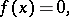(1)

whereis a differentiable function. The successive approximations of Newton's method are computed by the formulas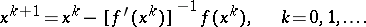(2)

Ifis twice continuously differentiable,is a simple root of (1) and the initial approximation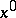lies sufficiently close to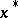, then Newton's method has quadratic convergence, that is,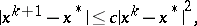whereis a constant depending only onand the initial approximation.

Frequently, for the solution of (1) one applies instead of (2) the so-called modified Newton method: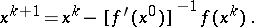(3)

Under the same assumptions under which Newton's method has quadratic convergence, the method (3) has linear convergence, that is, it converges with the rate of a geometric progression with denominator less than 1.

In connection with solving a non-linear operator equation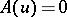with an operator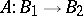, whereandare Banach spaces, a generalization of (2) is the Newton–Kantorovich method. Its formulas are of the form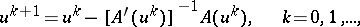where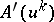is the Fréchet derivative ofat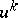, which is an invertible operator acting from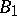to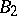. Under special assumptions the Newton–Kantorovich method has quadratic convergence, and the corresponding modified method has linear convergence (cf. also Kantorovich process).

I. Newton worked out his method in 1669.